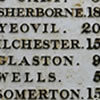#### You may also like### Whole Numbers Only

Can you work out how many of each kind of pencil this student bought?### Squaring the Circle

Bluey-green, white and transparent squares with a few odd bits of shapes around the perimeter. But, how many squares are there of each type in the complete circle? Study the picture and make an estimate.### Where Am I?

From the information you are asked to work out where the picture was taken. Is there too much information? How accurate can your answer be?

# Interstellar

##### Age 11 to 14 Short Challenge Level:

Answer: $19$

$\tfrac x5$ is between $3$ and $4$
$\therefore x$ is between $15$ and $20$
So $x=19$

This problem is taken from the UKMT Mathematical Challenges.
You can find more short problems, arranged by curriculum topic, in our short problems collection.